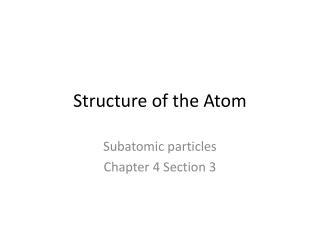DownloadDownload PresentationStructure of the Atom

# Structure of the Atom

Download Presentation## Structure of the Atom

- - - - - - - - - - - - - - - - - - - - - - - - - - - E N D - - - - - - - - - - - - - - - - - - - - - - - - - - -
##### Presentation Transcript

1. Structure of the Atom Subatomic particles Chapter 4 Section 3

2. Subatomic particles • Electron • Thomson’s cathode ray tube experiments • Charge of -1 • Mass is so small we can ignore it • Proton • Rutherford’s gold foil experiment • Charge of +1 • Mass = 1 amu • Neutron • Discovered by Chadwick • No charge (neutral) • Mass = 1 amu

3. Elemental particles • Electron • Quark

4. Quarks • Six flavors • Up • Down • Charm • Strange • Top (truth) • Bottom (beauty)

5. up and down quarks • Up quarks have a +2/3 charge • Down quarks have a -1/3 charge

6. Protons and neutrons • Protons are made up of two up quarks and one down • 2/3 + 2/3 + -1/3 = +1 • Neutrons are made up of one up quark and two down quarks • 2/3 + -1/3 + -1/3 = 0

7. Atomic number • Number of protons in an atom of an element • Atoms have no net charge (electrically = 0) so number of protons = number of electrons • Example: oxygen has 8 protons (+8) and 8 electrons (-8) • (+8) + (-8) = 0, no charge

8. Practice • Do practice problems 11, 12, and 13 p. 99

9. Mass number • The number of protons and neutrons in an atom’s nucleus added together • # protons + # neutrons = mass number • How could I calculate the number of neutrons in an atom’s nucleus?

10. Isotopes • Atoms of the same element that have different number of neutrons • Mass of an atom is made up of protons and neutrons • When number of neutrons changes, so does mass!!! • Proper notation for atoms/isotopes

11. Examples and Practice • Example p. 101 • Do # 14 p. 101

12. Atomic mass • Atomic mass unit = 1/12 of mass of carbon-12 atom • Atomic mass is the weighted average mass of the isotopes of that element • Example: chlorine has two isotopes, chlorine-35 (74%) and chlorine-37 (26%).

13. Ions • Ions are charged atoms. They have lost or gained electrons, so the number of electrons no longer equals the number of protons. • Example: O2- has gained two electrons protons (+8) + electrons (-10) = -2 charge